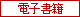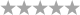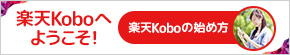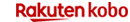トップ > 電子書籍 > 洋書 > Science & Nature > Mathematical Physics

# Zeros of Polynomials and Solvable Nonlinear Evolution Equations　（Cambridge University Press）　[電子書籍版] Francesco Calogero

• ユーザ評価0件）
•  | レビューを書く

10,729円（税込）## 商品説明

Reporting a novel breakthrough in the identification and investigation of solvable and integrable nonlinearly coupled evolution ordinary differential equations (ODEs) or partial differential equations (PDEs), this text includes practical examples throughout to illustrate the theoretical concepts. Beginning with systems of ODEs, including second-order ODEs of Newtonian type, it then discusses systems of PDEs, and systems evolving in discrete time. It reports a novel, differential algorithm which can be used to evaluate all the zeros of a generic polynomial of arbitrary degree: a remarkable development of a fundamental mathematical problem with a long history. The book will be of interest to applied mathematicians and mathematical physicists working in the area of integrable and solvable non-linear evolution equations; it can also be used as supplementary reading material for general applied mathematics or mathematical physics courses.

まだレビューがありません。

## 楽天ブックスランキング情報

•週間ランキング

ランキング情報がありません。

•日別ランキング

ランキング情報がありません。

## ご購入はこちらから電子書籍版

10,729円（税込）

2018/3/25(日) 23:59まで

エントリー＆楽天カード利用で
ポイント5倍

2018/3/30(金) 23:59まで
25,000円以上購入で使える！
40%OFF
5と0のつく日は楽天Koboがお得に！

## 最近チェックした商品

※表示を削除したい場合は閲覧履歴のページから削除お願いいたしますこのページの先頭へ